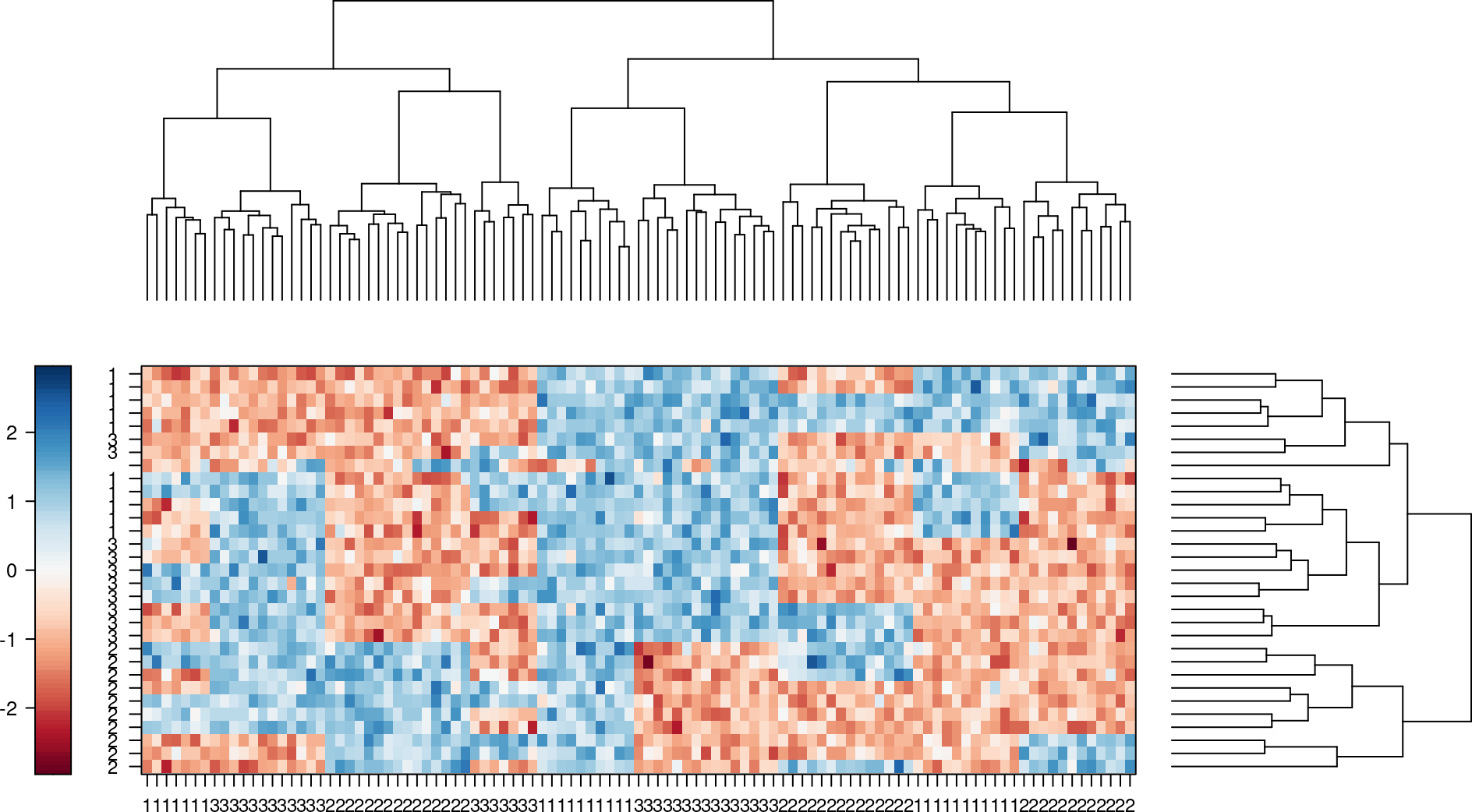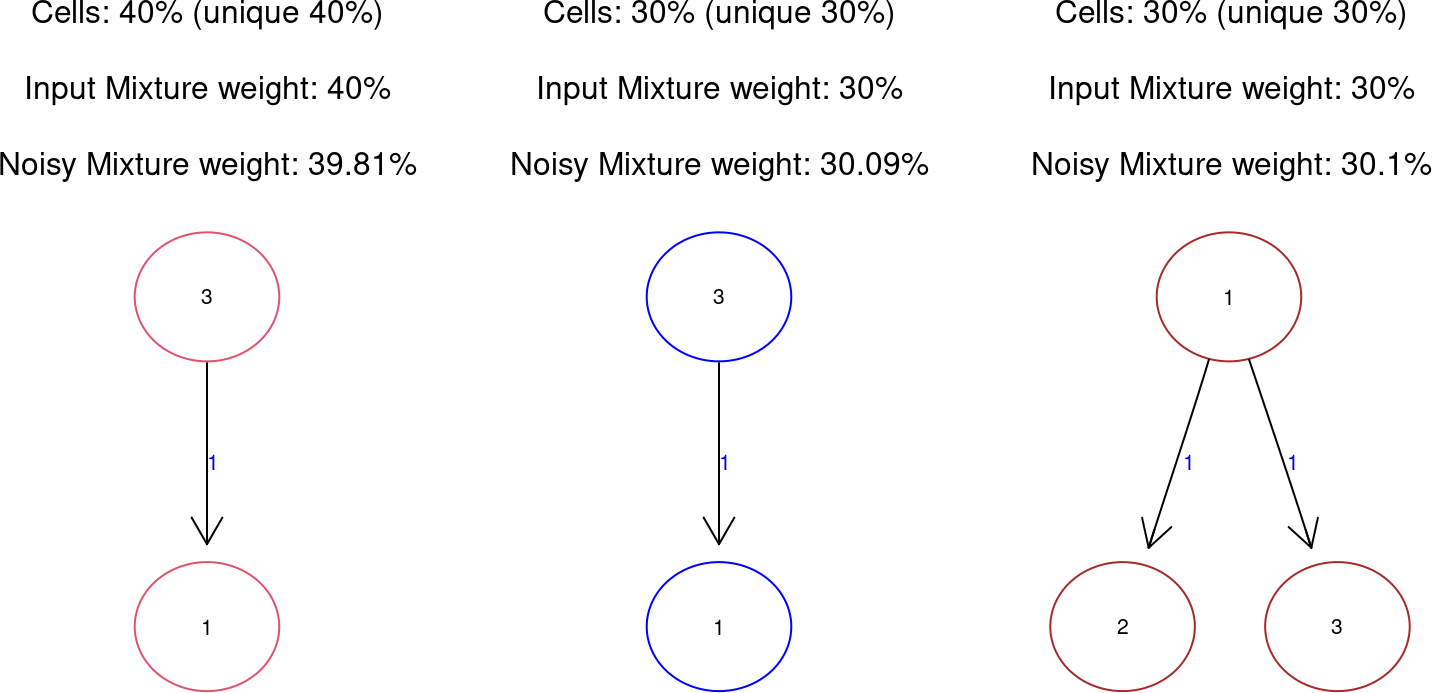# 1 Introduction

Single cell RNA-seq data sets from pooled CrispR screens provide the possibility to analyze heterogeneous cell populations. We extended the original Nested Effects Models (NEM) to Mixture Nested Effects Models (M&NEM) to simultaneously identify several causal signaling graphs and corresponding subpopulations of cells. The final result will be a soft clustering of the perturbed cells and a causal signaling graph, which describes the interactions of the perturbed signaling genes (S-genes) for each cluster of cells and the sub-topology for the observed genes (E-genes).

The M&NEM algorithm uses an expectation maximization (EM) algorithm to infer an optimum for \(k\) components. In the E-step the expectation of the hidden data (assignment of a cell to a component aka responsibilities) is calculated. Based on the responsibilities M&NEM weights the data for each component and the standard NEM approach is used to optimize the causal network for each weighted data set (M-step).

Install the package with the bioconductor manager package.

``````if (!requireNamespace("BiocManager", quietly = TRUE)) install.packages("BiocManager")
BiocManager::install("mnem")``````

Load the package with the library function.

``library(mnem)``

# 3 Small example

The input data is an m times n matrix of log odds (e.g. for fold changes as in the R package ‘limma’, Ritchie et al., 2015). The ith row of the jth column are the log odds for feature (E-gene) i in cell j. As in the case of the original NEM, the data consists of a multitude of E-genes. However, where for the original NEM only one column complies to each perturbed signaling gene (S-gene), M&NEM is designed for single cell data and therefore can handle and draws its power from multiple cells for each perturbed S-gene.

## 3.1 Data simulation

The M&NEM package includes a functions for the simulation of typical single cell log odds. We use this function to create data for three S-genes. Several E-genes for each S-gene including a small number of uninformative E-genes. Additionally we generate the data from a mixture of three components and sample a population of cells with different numbers of cells belonging to each component (approx. mixture weights: mw). The function simulates discrete data (\(1\) for effect and \(0\) for no effect). We transform the discrete data to log odds by adding Gaussian noise with mean \(1\) to all \(1\)s and with mean \(-1\) to all \(0\)s. Hint: If you use the ‘mw’ parameter, the ‘Nems’ parameter is not necessary.

Figure 1 shows a heatmap of our generated data. Since we used only mild noise, effects and no effects are still clearly distinguished into shades of blue and red. We can identify the uninformative E-gene (no rownames) by its random/unique patterns. There is a clear clustering noticeable for the knock-downs hinting at differential causal regulation within the cell population. The E-gene numbers denote the attachment of the E-genes in regards to the first component. For the other components attachments differ and do not agree with the E-gene name anymore.

``````seed <- 2
Sgenes <- 3
Egenes <- 10
nCells <- 100
uninform <- 1
mw <- c(0.4, 0.3, 0.3)
Nems <- 3
noise <- 0.5
set.seed(seed)
simmini <- simData(Sgenes = Sgenes, Egenes = Egenes, Nems = Nems, mw = mw, nCells = nCells,
uninform = uninform)
data <- simmini\$data
ones <- which(data == 1)
zeros <- which(data == 0)
data[ones] <- rnorm(length(ones), 1, noise)
data[zeros] <- rnorm(length(zeros), -1, noise)
epiNEM::HeatmapOP(data, col = "RdBu", cexRow = 0.75, cexCol = 0.75, bordercol = "transparent",
xrot = 0, dendrogram = "both")``````Figure 1: Heatmap of the simulated log odds
Effects are blue and no effects are red. Rows denote the observed E-genes and columns the S-genes. Each S-gene has been perturbed in many cells. The E-genes are annotated as how they are attached in the ground truth. E.g. E-genes named ‘1’ are attached to S-gene ‘1’ in the ground truth.

``plot(simmini, data)``Figure 2: Ground truth causal networks of our simulated mixture of cells

Figure 2 shows three different causal networks for the population of simulated cells. The chosen mixture weights almost agree with the noisy mixture weights due to only little noise.

## 3.2 Network inference w.r.t. complexity

We forget the solution stored in ‘simmini\$Nem’ and use the ‘mnem’ function to infer the network. We choose our a greedy search for the M-step and \(3\) independent starts for the EM algorithm to keep this vignette short. However, even for only three S-genes more starts are generally recommended.

Since the number of components \(k\) is a priori not known, we perform optimization for \(k=1,2,3,4\) and use our penalized log likelihood to choose the best \(k\). Figure 3 shows the best mixture of our causal networks with \(k=3\). We have found the same causal networks we used to generate the data.

We can speed up the computation with the ‘parallel = n’ parameter with \(n\) threads.

``````starts <- 5
bestk <- mnemk(data, ks = 1:5, search = "greedy", starts = starts, parallel = NULL)
plot(bestk\$best)``````